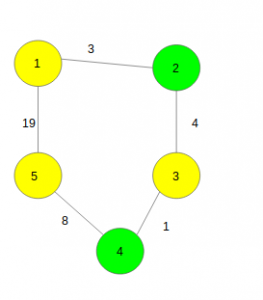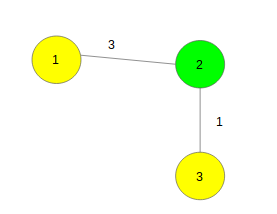# Find the shortest distance between any pair of two different good nodes

Given a weighted undirected connected graph with N nodes and M edges. Some of the nodes are marked as good. The task is to find the shortest distance between any pair of two different good nodes.

Note: Nodes marked as yellow in the below examples are considered to be good nodes.

Examples:

```Input :Output : 7
Explanation :
Pairs of Good Nodes and distance between them are:
(1 to 3) -> distance: 7,
(3 to 5) -> distance: 9,
(1 to 5) -> distance: 16,
out of which 7 is the minimum.

Input :Output : 4
```

## Recommended: Please try your approach on {IDE} first, before moving on to the solution.

Approach : Let us start by thinking of an algorithm to solve a simpler version of the given problem wherein all edges are of weight 1.

• Pick a random good node and perform a BFS from this point and stop at the first level saywhich contains another good node.
• We know that the minimum distance between any two good nodes can’t be more than s. So we again take a good node at random which is not already taken before and perform a BFS again. If we don’t find any special node in s distance, we terminate the search. If we do, then we update the value of s and repeat the procedure with some other special node taken at random.

We can apply a similar algorithm when weights are multiple.

Below is the implementation of the above approach:

 `// CPP program to find the shortest pairwise ` `// distance between any two different good nodes. ` `#include ` `using` `namespace` `std; ` ` `  `#define N 100005 ` `const` `int` `MAXI = 99999999; ` ` `  `// Function to add edges ` `void` `add_edge(vector > gr[], ``int` `x, ` `              ``int` `y, ``int` `weight) ` `{ ` `    ``gr[x].push_back({ y, weight }); ` `    ``gr[y].push_back({ x, weight }); ` `} ` ` `  `// Function to find the shortest ` `// distance between any pair of ` `// two different good nodes ` `int` `minDistance(vector > gr[], ``int` `n, ` `                ``int` `dist[], ``int` `vis[], ``int` `a[], ``int` `k) ` `{ ` `    ``// Keeps minimum element on top ` `    ``priority_queue, vector >, ` `                   ``greater > > q; ` ` `  `    ``// To keep required answer ` `    ``int` `ans = MAXI; ` ` `  `    ``for` `(``int` `i = 1; i <= n; i++) { ` `        ``// If it is not good vertex ` `        ``if` `(!a[i]) ` `            ``continue``; ` ` `  `        ``// Keep all vertices not visited ` `        ``// and distance as MAXI ` `        ``for` `(``int` `j = 1; j <= n; j++) { ` `            ``dist[j] = MAXI; ` `            ``vis[j] = 0; ` `        ``} ` ` `  `        ``// Distance from ith vertex to ith is zero ` `        ``dist[i] = 0; ` ` `  `        ``// Make queue empty ` `        ``while` `(!q.empty()) ` `            ``q.pop(); ` ` `  `        ``// Push the ith vertex ` `        ``q.push({ 0, i }); ` ` `  `        ``// Count the good vertices ` `        ``int` `good = 0; ` ` `  `        ``while` `(!q.empty()) { ` `            ``// Take the top element ` `            ``int` `v = q.top().second; ` ` `  `            ``// Remove it ` `            ``q.pop(); ` ` `  `            ``// If it is already visited ` `            ``if` `(vis[v]) ` `                ``continue``; ` `            ``vis[v] = 1; ` ` `  `            ``// Count good vertices ` `            ``good += a[v]; ` ` `  `            ``// If distance from vth vertex ` `            ``// is greater than ans ` `            ``if` `(dist[v] > ans) ` `                ``break``; ` ` `  `            ``// If two good vertices are found ` `            ``if` `(good == 2 and a[v]) { ` `                ``ans = min(ans, dist[v]); ` `                ``break``; ` `            ``} ` ` `  `            ``// Go to all adjacent vertices ` `            ``for` `(``int` `j = 0; j < gr[v].size(); j++) { ` `                ``int` `to = gr[v][j].first; ` `                ``int` `weight = gr[v][j].second; ` ` `  `                ``// if distance is less ` `                ``if` `(dist[v] + weight < dist[to]) { ` `                    ``dist[to] = dist[v] + weight; ` `                    ``q.push({ dist[to], to }); ` `                ``} ` `            ``} ` `        ``} ` `    ``} ` ` `  `    ``// Return the required answer ` `    ``return` `ans; ` `} ` ` `  `// Driver code ` `int` `main() ` `{ ` `    ``// Number of vertices and edges ` `    ``int` `n = 5, m = 5; ` ` `  `    ``vector > gr[N]; ` ` `  `    ``// Function call to add edges ` `    ``add_edge(gr, 1, 2, 3); ` `    ``add_edge(gr, 1, 2, 3); ` `    ``add_edge(gr, 2, 3, 4); ` `    ``add_edge(gr, 3, 4, 1); ` `    ``add_edge(gr, 4, 5, 8); ` ` `  `    ``// Number of good nodes ` `    ``int` `k = 3; ` ` `  `    ``int` `a[N], vis[N], dist[N]; ` ` `  `    ``// To keep good vertices ` `    ``a = a = a = 1; ` ` `  `    ``cout << minDistance(gr, n, dist, vis, a, k); ` ` `  `    ``return` `0; ` `} `

Output:

```7
```My Personal Notes arrow_drop_upCheck out this Author's contributed articles.

If you like GeeksforGeeks and would like to contribute, you can also write an article using contribute.geeksforgeeks.org or mail your article to contribute@geeksforgeeks.org. See your article appearing on the GeeksforGeeks main page and help other Geeks.

Please Improve this article if you find anything incorrect by clicking on the "Improve Article" button below.